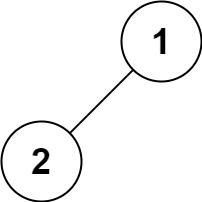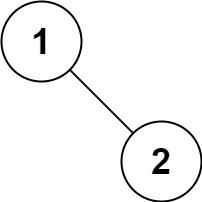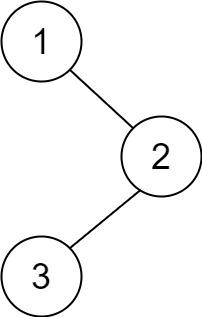2023-02-2300

1. 二叉树的前序遍历
1.1 题目描述
1.2 解法一：递归
1.3 解法二：迭代
2. 二叉树的后序遍历
2.1 题目描述
2.2 解法一：递归
2.3 解法二：迭代

# 1. 二叉树的前序遍历

## 1.1 题目描述``````输入： root = [1,null,2,3]

``````

``````输入： root = []

``````

``````输入： root = 

````````````输入： root = [1,2]

````````````输入： root = [1,null,2]

``````

• 树中节点数目在范围 `[0, 100]` 内
• `-100 <= Node.val <= 100`

## 1.2 解法一：递归

1. 优先访问根节点
2. 之后访问左子树
3. 最后访问右子树

```ts```function preorderTraversal(root: TreeNode | null): number[] {
let ret: number[] = [];
function traversal(root: TreeNode | null) {
if(!root) return
root.val !== null && ret.push(root.val);
traversal(root.left);
traversal(root.right);
}
traversal(root);

return ret;
}
``````

• 时间复杂度：`O(n)`，其中 `n` 是二叉树的节点数。每一个节点恰好被遍历一次。
• 空间复杂度：`O(n)`，为递归过程中栈的开销，平均情况下为 `O(logn)`，最坏情况下树呈现链状，为 `O(n)`

## 1.3 解法二：迭代

```ts```function preorderTraversal(root: TreeNode | null): number[] {
let ret: number[] = [];

if (!root) return [];
let stack: TreeNode[] = [root];

while (stack.length) {
const node = stack.pop()!;
if (node.val !== null) ret.push(node.val);
node.right && stack.push(node.right);
node.left && stack.push(node.left);
}

return ret;
}
``````

# 2. 二叉树的后序遍历

## 2.1 题目描述``````输入： root = [1,null,2,3]

``````

``````输入： root = []

``````

``````输入： root = 

``````

• 树中节点的数目在范围 `[0, 100]` 内
• `-100 <= Node.val <= 100`

## 2.2 解法一：递归

1. 优先访问左子树
2. 之后访问右子树
3. 最后访问根节点

```ts```function postorderTraversal(root: TreeNode | null): number[] {
let ret: number[] = [];
function traversal(root: TreeNode | null) {
if(!root) return
traversal(root.left);
traversal(root.right);
root.val !== null && ret.push(root.val);
}
traversal(root);

return ret;
}
``````

• 时间复杂度：`O(n)`，其中 `n` 是二叉树的节点数。每一个节点恰好被遍历一次。
• 空间复杂度：`O(n)`，为递归过程中栈的开销，平均情况下为 `O(logn)`，最坏情况下树呈现链状，为 `O(n)`

## 2.3 解法二：迭代

```ts```function postorderTraversal(root: TreeNode | null): number[] {
let ret: number[] = [];
let stack: TreeNode[] = [];
let current: TreeNode | null = root;
let lastVisitedNode: TreeNode | null = null;
while (stack.length || current !== null) {
while (current !== null) {
stack.push(current);
current = current.left;
}
current = stack[stack.length - 1];
if (current.right === null || current.right === lastVisitedNode) {
ret.push(current.val);
lastVisitedNode = current;
stack.pop();
current = null;
} else {
current = current.right;
}
}

return ret;
}
``````

• 时间复杂度：`O(n)`，其中 `n` 是二叉树的节点数。每一个节点恰好被遍历一次。
• 空间复杂度：`O(n)`，为递归过程中栈的开销，平均情况下为 `O(logn)`，最坏情况下树呈现链状，为 `O(n)`